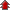Home Board Paper Solutions Text Book Solutions Articles NCERT Exemplar Solutions

# Chemical Bonding and Molecular Structure### Exercise 1

•  Q1 Explain the formation of a chemical bond. Q2 Write Lewis dot symbols for atoms of the following elements: Mg, Na, B, O, N, Br. Q3 Write Lewis symbols for the following atoms and ions: S and S2–; Al and Al3+; H and H– Q4 , HCOOH">Draw the Lewis structures for the following molecules and ions: H2S, SiCl4, BeF2,, HCOOH Q5 Define octet rule. Write its significance and limitations. Q6 Write the favourable factors for the formation of ionic bond. Q7 Discuss the shape of the following molecules using the VSEPR model: BeCl2, BCl3, SiCl4, AsF5, H2S, PH3 Q8 Although geometries of NH3 and H2O molecules are distorted tetrahedral, bond angle in water is less than that of ammonia. Discuss. Q9 How do you express the bond strength in terms of bond order? Q10 Define the bond length. Q11 ion.">Explain the important aspects of resonance with reference to theion. Q12 H3PO3 can be represented by structures 1 and 2 shown below. Can these two structures be taken as the canonical forms of the resonance hybrid representing H3PO3? If not, give reasons for the same.">H3PO3 can be represented by structures 1 and 2 shown below. Can these two structures be taken as the canonical forms of the resonance hybrid representing H3PO3? If not, give reasons for the same.Q13 .">Write the resonance structures for SO3, NO2 and. Q14 Use Lewis symbols to show electron transfer between the following atoms to form cations and anions: (a) K and S (b) Ca and O (c) Al and N. Q15 Although both CO2 and H2O are triatomic molecules, the shape of H2O molecule is bent while that of CO2 is linear. Explain this on the basis of dipole moment. Q16 Write the significance/applications of dipole moment. Q17 Define electronegativity. How does it differ from electron gain enthalpy? Q18 Explain with the help of suitable example polar covalent bond. Q19 Arrange the bonds in order of increasing ionic character in the molecules: LiF, K2O, N2, SO2 and ClF3. Q20 ">The skeletal structure of CH3COOH as shown below is correct, but some of the bonds are shown incorrectly. Write the correct Lewis structure for acetic acid.Q21 Apart from tetrahedral geometry, another possible geometry for CH4 is square planar with the four H atoms at the corners of the square and the C atom at its centre. Explain why CH4 is not square planar? Q22 Explain why BeH2 molecule has a zero dipole moment although the Be–H bonds are polar. Q23 Which out of NH3 and NF3 has higher dipole moment and why? Q24 What is meant by hybridisation of atomic orbitals? Describe the shapes of sp, sp2, sp3 hybrid orbitals. Q25 ">Describe the change in hybridisation (if any) of the Al atom in the following reaction.Q26 Is there any change in the hybridisation of B and N atoms as a result of the following reaction? BF3 + NH3 → F3B.NH3 Q27 Draw diagrams showing the formation of a double bond and a triple bond between carbon atoms in C2H4 and C2H2 molecules. Q28 What is the total number of sigma and pi bonds in the following molecules? (a) C2H2 (b) C2H4 Q29 Considering x-axis as the internuclear axis which out of the following will not form a sigma bond and why? (a) 1s and 1s (b) 1s and 2px (c) 2py and 2py (d) 1s and 2s. Q30 Which hybrid orbitals are used by carbon atoms in the following molecules? (a)CH3–CH3; (b) CH3–CH=CH2; (c) CH3-CH2-OH; (d) CH3-CHO (e) CH3COOH Q31 What do you understand by bond pairs and lone pairs of electrons? Illustrate by giving one example of each type. Q32 Distinguish between a sigma and a pi bond. Q33 Explain the formation of H2 molecule on the basis of valence bond theory. Q34 Write the important conditions required for the linear combination of atomic orbitals to form molecular orbitals. Q35 Use molecular orbital theory to explain why the Be2 molecule does not exist. Q36 Compare the relative stability of the following species and indicate their magnetic properties: O2,O2+,O2- (superoxide), O22-(peroxide)">Compare the relative stability of the following species and indicate their magnetic properties: O2,O2+,O2- (superoxide), O22-(peroxide) Q37 Write the significance of a plus and a minus sign shown in representing the orbitals. Q38 Describe the hybridisation in case of PCl5. Why are the axial bonds longer as compared to equatorial bonds?">Describe the hybridisation in case of PCl5. Why are the axial bonds longer as compared to equatorial bonds? Q39 Define hydrogen bond. Is it weaker or stronger than the van der Waals forces? Q40 What is meant by the term bond order? Calculate the bond order of: N2, O2,O2+,and O2-.